PSABM4X8 - Practice---Acc7.3 Lesson 4 Reasoning about Equations and Tape Diagrams (Part 1) (6.EE.B.5, 7.EE.B.3, 7.EE.B.4.a)
Part A)

Here are three stories:

A. A family buys 6 tickets to a show. They also pay a $3 parking fee. They spend$27 to see the show.

B. Diego has 27 ounces of juice. He pours equal amounts for each of his 3 friends and has 6 ounces left for himself.

C. Jada works for 6 hours preparing for the art fair. She spends 3 hours on a sculpture and then paints 27 picture frames.

Here are three equations:

1.2.3.Decide which equation represents each story.

Which equation represents story A?

Select one:
Part B)

What does x represent?

Part C)

Find the solution.

Type your answer below as a number (example: 5, 3.1, 4 1/2, or 3/2):
Part D)

Explain or show your reasoning using the "WIRIS editor" button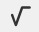.

Part E)

What does the solution tell you about the situation?

Part F)

Which equation represents story B?

Select one:
Part G)

What does x represent?

Part H)

Find the solution.

Type your answer below as a number (example: 5, 3.1, 4 1/2, or 3/2):
Part I)

Explain or show your reasoning using the "WIRIS editor" button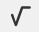.

Part J)

What does the solution tell you about the situation?

Part K)

Which equation represents story C?

Select one:
Part L)

What does x represent?

Part M)

Find the solution.

Type your answer below as a number (example: 5, 3.1, 4 1/2, or 3/2):
Part N)

Explain or show your reasoning using the "WIRIS editor" button.

Part O)

What does the solution tell you about the situation?

Part A)

Here is a diagram and its corresponding equation. Find the solution to the equation.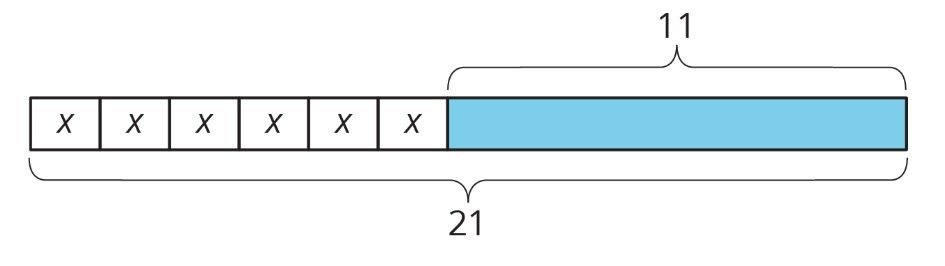6x + 11 = 21

What is the solution?

Type your answer below as a number (example: 5, 3.1, 4 1/2, or 3/2):
Part B)

For each object, choose an appropriate scale for a drawing that fits on a regular sheet of paper. Not all of the scales on the list will be used.

For your answer, write it out like this: paper: 1 in : 1ft.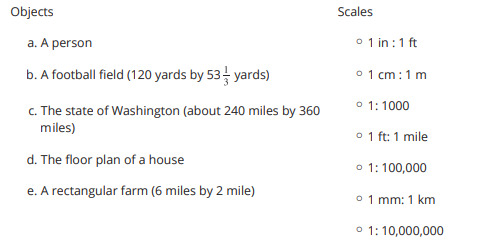Part A)

The diagram shows two intersecting lines. Find the missing angle measures.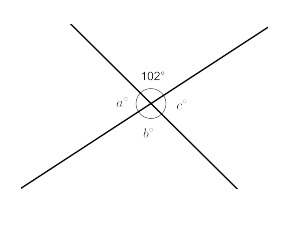a=

Type your answer below as a number (example: 5, 3.1, 4 1/2, or 3/2):
Part B)

The diagram shows two intersecting lines. Find the missing angle measures.bType your answer below as a number (example: 5, 3.1, 4 1/2, or 3/2):
Part C)

The diagram shows two intersecting lines. Find the missing angle measures.c